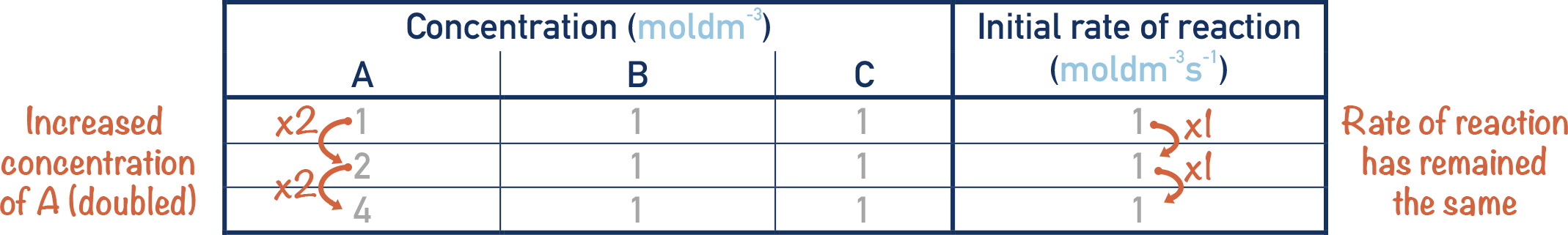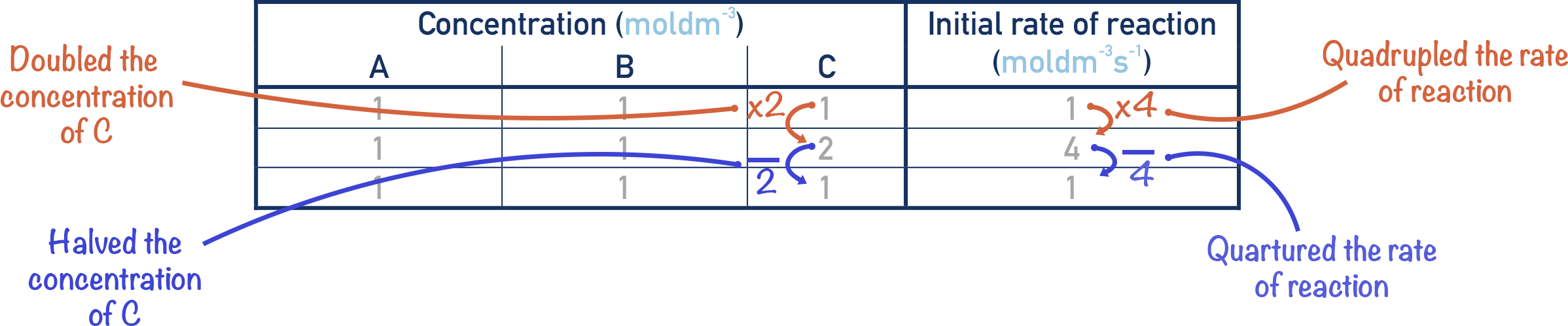Video Tutorial Orders of Reaction

Quick Notes Orders of Reaction

• Changing the concentration of reactants in a reaction can affect the rate of the reaction.
• The orders of a reaction describe how much the rate of a reaction changes when the concentrations of reactants are changed.
• The order of a reaction with respect to a reactant describes how much the rate of a reaction changes when the concentration of that reactant is changed by a given factor.
• Zero order means changing the concentration of the reactant has no effect on the rate of reaction.
• First order means changing the concentration of the reactant by a given factor will change the rate of the reaction by the same factor.
• Second order means changing the concentration of the reactant by a given factor will change the rate of the reaction by the same factor squared (x2).

Full Notes Orders of Reactions

The rate of a reaction is a measure of how fast a reaction is occurring. Changing the concentrations of reactants in a reaction can alter how fast the reaction happens, but not always by the factor we have changed the concentration by. For example, sometimes a reactant's concentration is doubled and yet the reaction rate doesn’t change at all!

How much the rate of a reaction is determined by the concentrations of its reactants is described by its ‘order of reaction’.

Orders of a reaction just link changes in concentrations of reactants to changes in the rate of a reaction. The order of a reaction ‘with respect to…’ just means how changing the concentration of a particular reactant affects the rate of the reaction (independent of other reactants).

There are three types of orders you need to know for A-level Chemistry – zero order, first order and second order.

For example, in the following reaction:Let’s say we are measuring the rate of this reaction, and we want to see how changing the concentrations of each reactant affects the overall rate.

## Zero Order

If we double the concentration of A and the rate does not change, then the order of the reaction with respect to A is zero order.It does not matter what we change the concentration of A to, the rate will always be the same.

## First Order

If we double the concentration of B and the rate also doubles, then the order of the reaction with respect to B is first order.Whatever factor we change the concentration of B by, the rate will change by the same factor (i.e. doubling the concentration of B results in a doubling of the rate, halving the concentration of B results in a halving of the rate, and so on).

## Second Order

If we double the concentration of C, and the rate quadruples, then the order of the reaction with respect to C is second order.Here, the rate of a reaction is changed by a factor that is the change in concentration squared. If we double the concentration of C, then the rate would change by 22 = 4. If we halved the concentration of C, then the rate would change by 0.52 = 0.25 (the rate would be quartered).